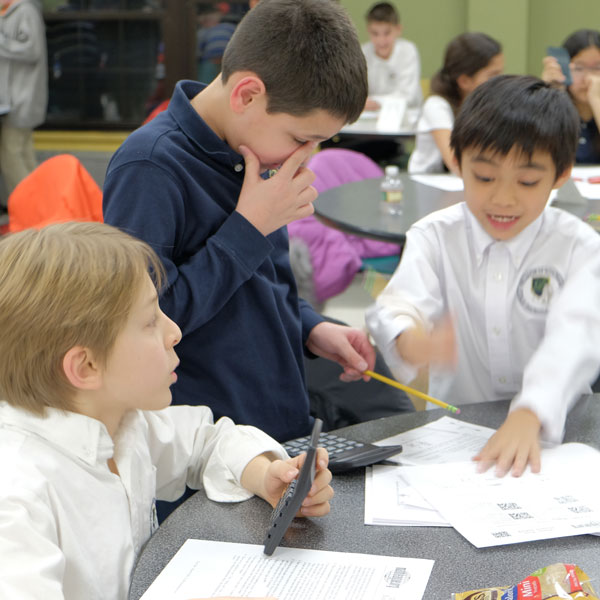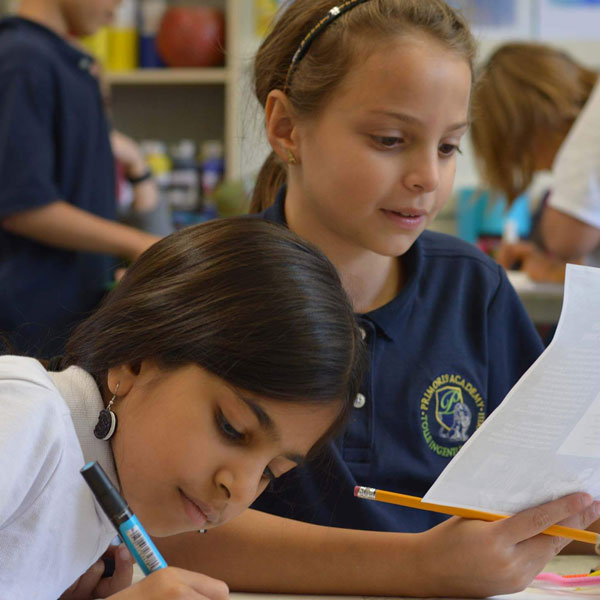# Math

With a strong focus on mental math and number sense concepts, Primoris Academy students are introduced to practical applications in mathematics at a young age. Through discussion, group work, and problem-solving, students will engage in mathematical concepts in both designed and real-life applications. Instruction is built for students to understand the WHY of math, rather than rote calculation sequences.

Students continually practice the following skills:

• Logical Reasoning
• Creative and Critical Thinking
• Multi-Step Complex Problem SolvingWhile practicing foundational skills, as well as divergent thinking, students continue their mathematical study through the following course sequence: Prealgebra, Algebra I, Geometry, Intermediate Algebra/Trigonometry, Precalculus, and Calculus.

In keeping with our overall school philosophy, students progress through the mathematical course of study based on their academic readiness and maturity. Students have the opportunity to engage in higher-level math topics such as Linear Algebra and Multivariable Calculus after completing the defined course of study.